# 2. Variables

 DEFINITION: A variable holds values which can change.

In order to set up a model, you need to be able to change parts of it in order to see what effect that has on the overall result.

This is what a variable can do for you - a variable allows you to change the model that then re-calculates the result.

The simplest kind of variable is a single cell containing a number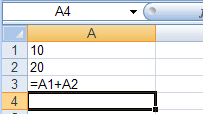Cell A1 and A2 are 'variables', because if you change their values, the result in A3 will change. So the simplest kind of variable is a single cell reference.

#### Named variable - why?

The formula shown above is so simple that it is easy to understand what it is doing. But things can get (and do) become far more complicated. For instance, consider a formula like this: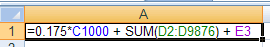It is much more difficult to understand the purpose of this formula. You can see that is seems to be adding up a range of cells and also multiplying a cell value but why?

Now lets set up the same formula in a slightly different way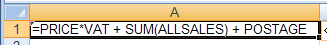It is still a little complicated but now it is much more clear as to what it is doing. In this case it is taking the price variable and VAT variable to work out the VAT on an item, then adds the postage costs. It then adds these to the total sales.

This can be done in a spreadsheet because it supports the idea of a 'Named variable'.

A named variable substitutes text for referencing a cell or range of cells.

#### Creating a Named variable

This is quite straight forward as it can be done right on screen.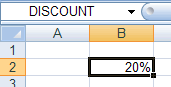Select the cell you want to use (B2)

In the top left box enter the name you want to use (DISCOUNT) and press enter.

That's it. You can now use DISCOUNT instead of cell B2 in a formula.

A Range of cells can also be given a single variable name, like this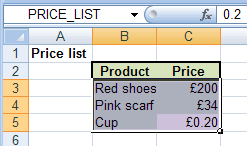Select the range of cells you want to set a name for. In this case the purple area is selected.

Type in a sensible name in the name box on the top left and press enter. By the way, you do not have to use capitals, lower case can be used as well - but no spaces, which is why I used an underscore to split the name.

Why would you want to name a range? Well, an extremely common way of using a spreadsheet is to 'look up' a value from a table. So a standard lookup formula may be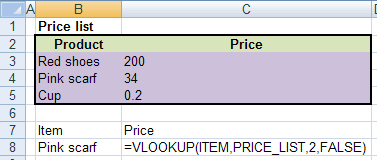In this case it is easy to see that the lookup formula is taking ITEM ( I set cell B8 as ITEM) and looking up its price in the PRICE_LIST table. I am sure you will agree that this is easier to understand than doing it without named variables as shown below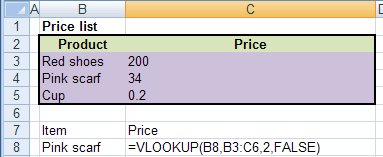challenge see if you can find out one extra fact on this topic that we haven't already told you